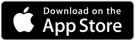# KQ MiniSynth – Modulators

### Curve Function (Version 3.1)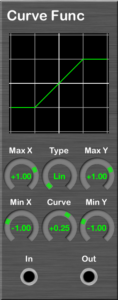Calcrate a fomula and output it. See the graph.
Input is X-axis (horizontal) and output is Y-axis (vertical).

#### Connectors / Parameters

• ##### Max X, Min X

Set the range of input. It flips horizontally when Max < Min.

• ##### Max Y, Min Y

Set the range of output. It flips vertically when Max < Min.

• ##### Type

Specify the following function type:

• Linear (Lin)
• Exponential (Exp)
• Logarithmic (Log)
• Point Symmetry Exponential (PSExp)
• Point Symmetry Logarithmic (PSLog)
• Symmetry Exponential (SExp)
• Symmetry Logarithmic (SLog)
• Switch (SW): Minimum when X < middle, Maximum when X > middle.
• ##### Curve

Specifies the amount of curve of the function. This is ignored if Type is Lin or SW.

• ##### In

Input source.

• ##### Out

Processed output.

### Multiplier (Version 3.1)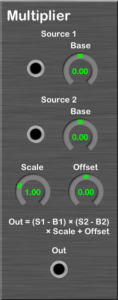Calculate multiplication.

This calculated as follows:
Out = (Source 1 – Base 1) × (Source 2 – Base 2) × Scale + Offset

#### Connectors / Parameters

The inputs.

• ##### Base

Set the base value of the source.

• ##### Scale

Multiplication scale.

The output.

### V/Oct Vibrator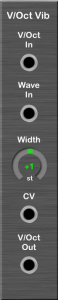This is a module which lets the input pitch vibrate and outputs it.
Try to connect LFO to “Wave In” and connect “V/Oct Out” to an Oscillator’s “V/Oct”.

#### Connectors/Parameters

• ##### V/Oct In

Select a pitch source to vibrate.

• ##### Wave In

Select a wave source contributing to vibrate.

• ##### Width

A width to vibrate.

• ##### CV

Select a source to input the amount of the width to vibrate.

• ##### V/Oct Out

This outputs a pitch.

### Keyboard Level Scaling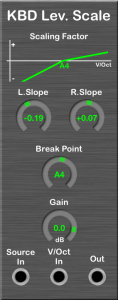This is a module which lets an input value increase/decrease by input pitch and outputs it.
The rate to increase/decrease is the value of “L.Slope” when input pitch is lower than “Break Point”, the value of “R.Slope” when the pitch is higher.

#### Connectors/Parameters

• ##### L.Slope/R.Slope

A rate to increase/decrease. A positive value to increase, a negative one to decrease.

• ##### Break Point

A threshold point.

• ##### Gain

A value for changing the output volume.

• ##### Source In

Select a source to process.

• ##### V/Oct In

Select a pitch source.

• ##### Out

This outputs the processed.

### Ring Modulator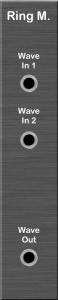This is a module to do “Ring-Modulation”.

#### Connectors/Parameters

• ##### Wave In 1,2

Select sources to input waves to do ring-modulation.

• ##### Out

This outputs the ring-modulated.

### Wave Shaper (Version 3.0)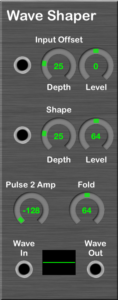This modifies the input wave’s shape. I recommend that the input be triangle wave.

#### Connectors / Parameters

• ##### Input Offset

Add this value to Wave In. The value is Level + Input × Depth.

• ##### Shape

Set to 0 when the absolute value of Wave In falls below this threshold. The value is Level + Input × Depth.

• ##### Pulse 2 Amp

Increase or decrease by this value if Wave In is less than 0.

• ##### Fold

Multiplied by this value and make the waveform folded when the Shape process is applied.

The input.

• ##### Wave Out

The output.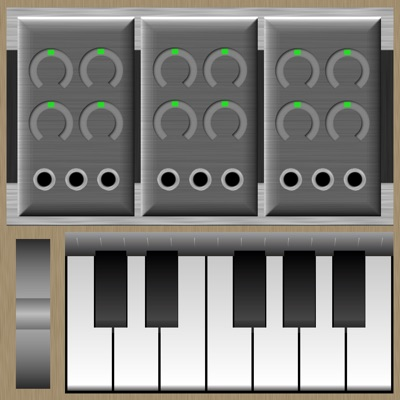KQ MiniSynth
Ryouta Kira